# SVM 透彻理解与分析

hit2015spring $\frac{hit2015spring}{晨凫追风}$

minw,b12w2 $\min\limits_{w,b}\qquad \frac 1 2 \|w\|^2$
s.t.yi(wTxi+b)1,i=1,2,,m $s.t.\qquad y_i(w^Tx_i+b)\geqslant 1, \qquad i=1,2,\cdots,m$

01yi(wTxi+b),i=1,2,,m $0\geqslant 1-y_i(w^Tx_i+b), i=1,2,\cdots,m$

L(w,b,α)=12w2+i=1mαi(1yi(wTxi+b)) $L(\mathbf{w},b,\alpha)=\frac 1 2 \|w\|^2+ \sum\limits_{i=1}^{m} \alpha_i (1-y_i(\mathbf{w^T x_i}+b))$

#### 对偶问题

maxα,xxL(x,α)(1)

s.t.xL(x,α)=0(2)

α>0(3)

minf(x)(4)

s.t.ci(x)0,i=1,2,3,p(5)

minf(x)s.t.c(x)=0c(x)0(6)

1、线性条件： p+q $p+q$个约束函数都是线性函数
2、梯度无关条件：梯度向量集 {ci(x¯)|iA¯} $\{\triangledown c_i(\bar x)|i \in \mathcal{\bar{A}}\}$线性无关，其中 A¯ $\mathcal{\bar{A}}$ x¯ $\bar x$处的有效集

（1）若原始问题 (4)(5) $(4)\sim (5)$有解，则它的Wolfe对偶问题 (1)(3) $(1)\sim(3)$有解
（2）若原始问题和它的Wolfe对偶问题分别有可行解 x¯α¯ $\bar x \text和 \bar \alpha$,则这两个解分别为原始问题和对偶问题最优解的充要条件是它们相应的原始问题和对偶问题的目标函数值相等

w=i=1mαiyixi0=i=1mαiyi(7)

12w2w2+i=1mαi+i=1mαiyib(8)

maxαi=1mαi12i=1mj=1mαiαjyiyjxTixjs.t.i=1mαiyi=0αi0,i=1,2,,m(9)

f(x)=wTx+b=i=1mαiyixTix+b(10)

αi0yif(xi)10αi(yif(xi)1)=0(11)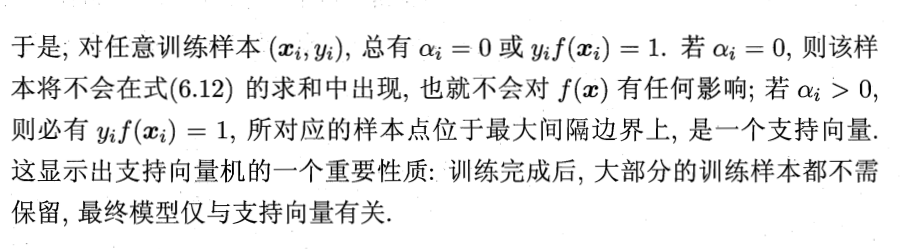### 线性支持向量机与软间隔

minw,b,ζ12w2+Ci=1mζi(12)

minw,b,ζ12w2+Ci=1mζis.t.yi(wxi+b)1ζiζi0,i=1,2,,m(13)

L(w,b,ζ,α,μ)=12w2+Ci=1mζii=1mαi(yi(wTxi+b)1+ζi)i=1mμiζi(14)

wL(w,b,ζ,α,μ)=wi=1mαiyixi=0 $\triangledown_w L(\mathbf{w},b,\zeta,\alpha,\mu)=w-\sum\limits_{i=1}^{m}\alpha_i y_i x_i=0$
bL(w,b,ζ,α,μ)=i=1mαiyi=0 $\triangledown_b L(\mathbf{w},b,\zeta,\alpha,\mu)=-\sum\limits_{i=1}^{m}\alpha_i y_i =0$
ζiL(w,b,ζ,α,μ)=Cαiμi=0 $\triangledown_{\zeta_i} L(\mathbf{w},b,\zeta,\alpha,\mu)=C-\alpha_i-\mu_i=0$

w=i=1mαiyixi(15)

i=1mαiyi=0(16)

Cαiμi=0(17)

minw,b,ζL(w,b,ζ,α,μ)=i=1mαi12i=1mj=1mαiαjyiyjxTixj(18)

maxαi=1mαi12i=1mj=1mαiαjyiyjxTixjs.t.i=1mαiyi=0Cαiμi=0αi0μi0,i=1,2,,m(19)

0αIC $0 \leq \alpha_I \leq C$

maxαi=1mαi12i=1mj=1mαiαjyiyjxTixjs.t.i=1mαiyi=00αiCi=1,2,,m(20)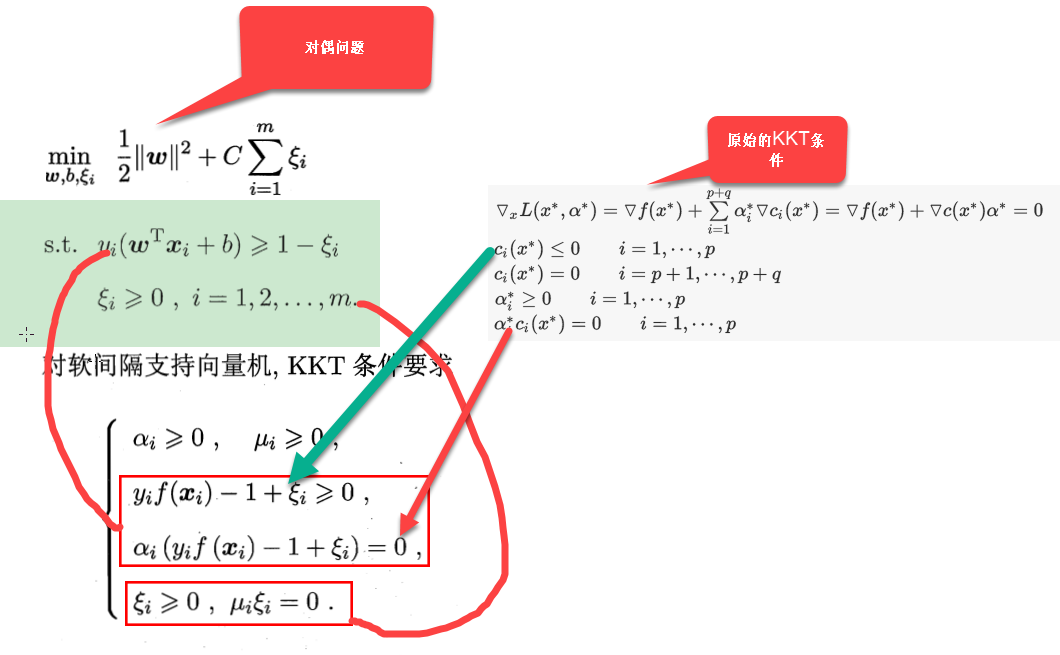#### 损失函数

0-1损失函数：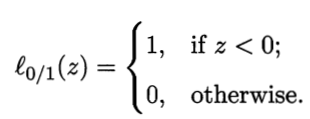minw,b,ζ12w2+Ci=1ml0/1(yi(wTxi+b)1)(21)

ϵ $\epsilon -$不敏感损失函数
hinge损失函数 ： l(z)=max[0,1z] $l(z)=\max [0,1-z]$,此时的优化函数为：
minw,b,ζ12w2+Ci=1mmax[0,1yi(wTxi+b)](22)

minw,b,ζ12w2+Ci=1mζis.t.yi(wxi+b)1ζiζi0,i=1,2,,m(23)

$\therefore \qquad \qquad$ 1yi(wTxi+b)>0 $1-y_i (w^T x_i+b)>0$
1yi(wTxi+b)=ζi $\qquad \qquad\qquad 1-y_i (w^T x_i+b) = \zeta_i$
$\qquad \qquad \qquad$ 1yi(wTxi+b)0 $1-y_i (w^T x_i+b)\leq0$
ζi=0 $\qquad \qquad \qquad \qquad \qquad \zeta_i=0$
1yi(wTxi+b)ζi $\therefore \qquad \qquad 1-y_i (w^T x_i+b)\leq \zeta_i$
yi(wTxi+b)1ζi $\therefore \qquad \qquad y_i (w^T x_i+b)\geq 1- \zeta_i$

s.t.yi(wxi+b)1ζi ζi0,i=1,2,,m $s.t. \qquad y_i(wx_i+b)\geq1-\zeta_i \ \qquad \qquad \zeta_i \geq 0,\qquad i = 1,2,\cdots,m$
$\therefore \qquad \qquad$ 最优化问题 minw,bλw2+i=1mmax[0,1yi(wTxi+b)] $\min\limits_{w,b} \qquad \lambda \|w\|^2 + \sum\limits_{i=1}^{m}\max [0,1-y_i(w^Tx_i+b)]$ 可以写成：

minw,bλw2+i=1mζi $\qquad \qquad \qquad \qquad\min\limits_{w,b} \qquad \lambda \|w\|^2 + \sum\limits_{i=1}^{m}\zeta_i$
λ=12C $\lambda = \frac 1{2C}$可以得到：
minw,b1C(12w2+Ci=1mζi) $\qquad \qquad \qquad \qquad\min\limits_{w,b} \qquad \frac 1 C(\frac 1 2\|w\|^2 +C \sum\limits_{i=1}^{m}\zeta_i)$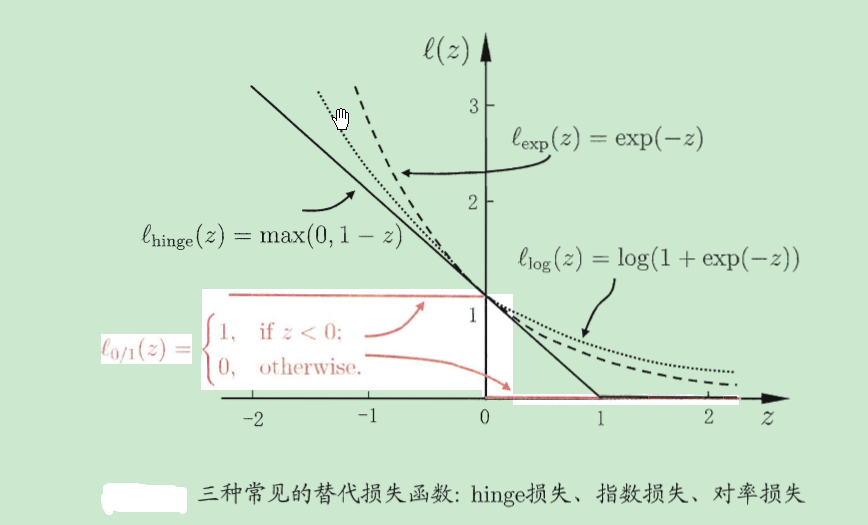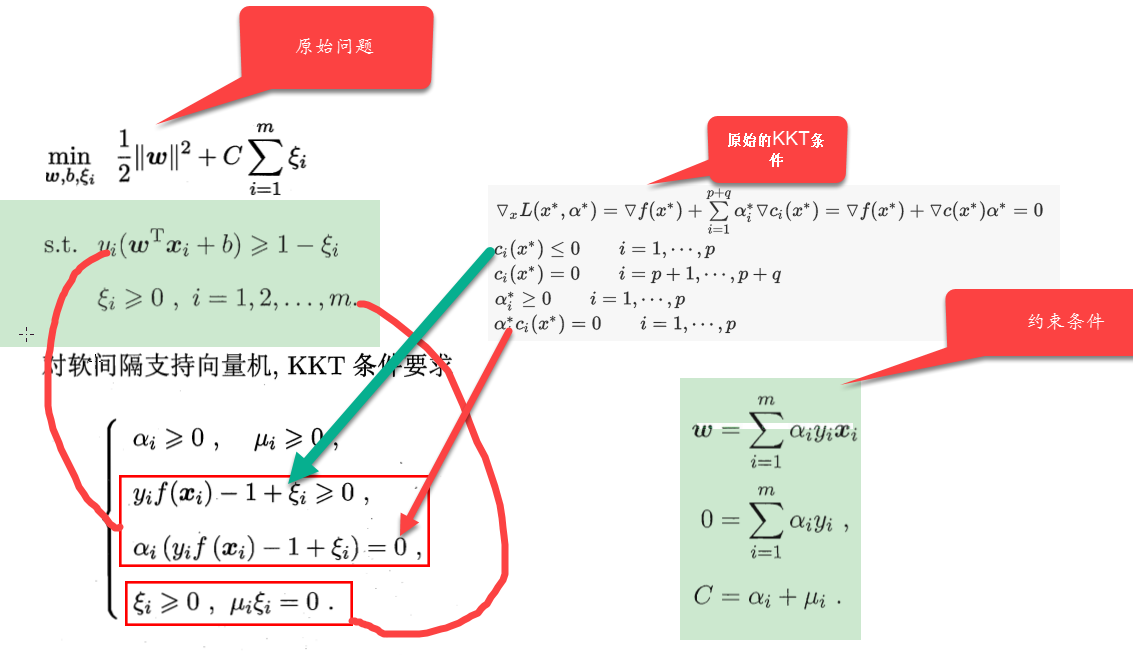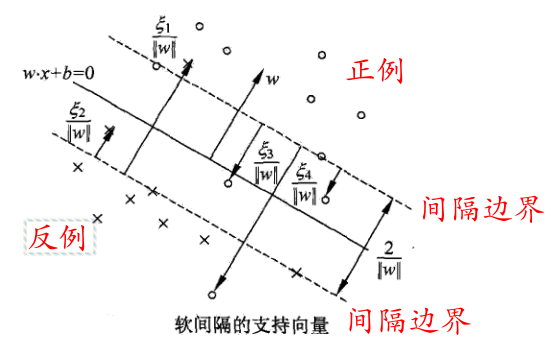1 $1^\circ$,当 0<αi<C $0<\alpha_i < C$时，此时由约束条件 C=αi+μi $C=\alpha_i+\mu_i$得到， μi>0 $\mu_i>0$, ζi=0 $\therefore \zeta_i =0$此时样本落在间隔的边界上面。这时候损失函数是0

2 $2^\circ$,当 αi=C $\alpha_i = C$时，此时由约束条件 C=αi+μi $C=\alpha_i+\mu_i$得到， μi=0 $\mu_i=0$, $\therefore$ ζi1 $\zeta_i \leq1$时，此时样本落在间隔的内部，即两个间隔之间。当 ζi>1 $\zeta_i>1$时，此时样本错误分类。这时候损失函数起作用，根据样本点的分类情况而定。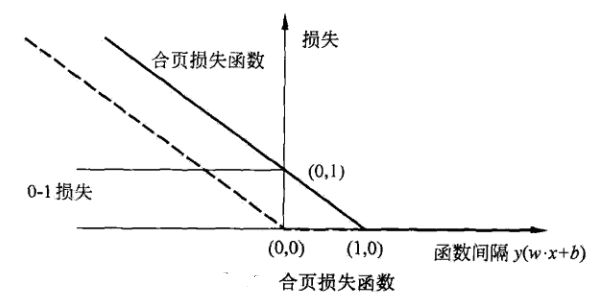minfΩ(f)+Ci=1ml(f(xi),yi)(24)

#### VC维概念的理解

VC维

vc维是度量一个假设集 F $\mathcal F$的表达能力的，对于VC维的定义：

(1) N(F,Zm) $\mathcal N (\mathcal F,Z_m)$:这个概念是表示：

1、 F $\mathcal F$是一个假设集，理解为决策函数的集合，它们在 XR $\mathcal X \in R$上取值为-1或者+1
2、 Zm=x1,,xm $Z_m={x_1,\cdots,x_m}$就是 X $\mathcal X$空间里面的m个点构成的集合

Zm $Z_m$中的每一个点代入 F $\mathcal F$中的一个函数 fm(x) $f_m(x)$，得到一个m维的向量（f(x_1,\cdots,f(x_m))），其实这个向量就是-1和+1构成的向量,当把 F $\mathcal F$里面的所有的 f $f$都取到后，所得到的不同的m维向量的个数就是N(F,Zm)$\mathcal N (\mathcal F,Z_m)$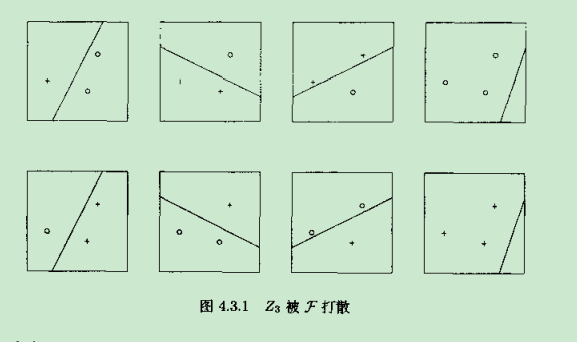F=f(x,α)=sgn(α2[x]2+α1[x]1+α0) $\mathcal F = {f(x,\alpha)=sgn (\alpha_2 [x]_2+\alpha_1 [x]_1+\alpha_0)}$

Z3=x1,x2,x3 $Z_3={x_1,x_2,x_3}$,上面的图中， Z3 $Z_3$ F $\mathcal F$打散，就是如上图所示，当取不同的 f $f$的时候，那三个点的决策值是不一样的值，可以取编23=8$2^3=8$种情况，这样就能说明， Zm $Z_m$ F $\mathcal F$打散

N(F,m)=maxN(F,Zm)|ZmX $\mathcal N(\mathcal F,m)=max{\mathcal N (\mathcal F,Z_m)| \qquad Z_m \in \mathcal X}$

VC维表示的是在空间 X $\mathcal X$中能打散的最多的点数。这里的最多是存在性问题。

#### 支持向量机的总体理解

07-081658
03-02328504-15114
08-20230
01-181106
07-028797
09-071651
08-102万+
12-202万+